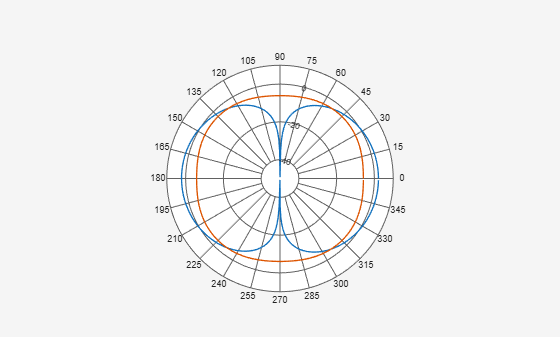Add data to polar plot

## Syntax

``add(p,d)``
``add(p,angle,magnitude)``

## Description

example

````add(p,d)` adds new antenna data to the polar plot, `p` based on the real amplitude values, `data`. ```

example

````add(p,angle,magnitude)` adds data sets of `angle` vectors and corresponding `magnitude` matrices to polar plot `p`.```

## Input Arguments

expand all

Polar plot, specified as a scalar handle.

Antenna or array data, specified as one of the following:

• A real length-M vector, where M contains the magnitude values with angles assumed to be $\frac{\left(0:M-1\right)}{M}×{360}^{\circ }$ degrees.

• A real M-by-N matrix, where M contains the magnitude values and N contains the independent data sets. Each column in the matrix has angles taken from the vector $\frac{\left(0:M-1\right)}{M}×{360}^{\circ }$ degrees. The set of each angle can vary for each column.

• A real N-D array, where N is the number of dimensions. Arrays with dimensions `2` and greater are independent data sets.

• A complex vector or matrix, where `data` contains Cartesian coordinates ((x,y) of each point. x contains the real part of `data` and y contains the imaginary part of `data`.

When data is in a logarithmic form such as dB, magnitude values can be negative. In this case,`polarpattern` plots the lowest magnitude values at the origin of the polar plot and highest magnitude values at the maximum radius.

Set of angles, specified as a vector in degrees.

Set of magnitude values, specified as a vector or a matrix. For a matrix of magnitude values, each column is an independent set of magnitude values and corresponds to the same set of angles.

## Examples

expand all

Create a helix antenna that has 28 mm radius, 1.2 mm width, and 4 turns. Calculate the directivity of the antenna at 1.8 GHz.

```hx = helix('Radius',28e-3,'Width',1.2e-3,'Turns',4); H = pattern(hx, 1.8e9,0,0:1:360);```

Plot the polar pattern.

`P = polarpattern(H);`Create a dipole antenna and calculate the directivity at 270 MHz.

```d = dipole; D = pattern(d,270e6,0,0:1:360);```

Add the directivity of the dipole to the existing polar plot of helix antenna.

`add(P,D);`Create a dipole and plot the polar pattern of its directivity at a frequency of 75 MHz.

```d = dipole; D = pattern(d,75e6,0,0:1:360); P = polarpattern(D);```Create a cavity antenna. Calculate the directivity of the antenna at 1 GHz. Write the directivity of the antenna to `cavity.pln` using the `msiwrite` function.

```c = cavity; msiwrite(c,1e9,'cavity','Name','Cavity Antenna Specifications');```

Read the data from `cavity.pln` to `Horizontal`, `Vertical`, and `Optional` structures using the `msiread` function.

`[Horizontal,Vertical,Optional] = msiread('cavity.pln')`
```Horizontal = struct with fields: PhysicalQuantity: 'Gain' Magnitude: [360x1 double] Units: 'dBi' Azimuth: [360x1 double] Elevation: 0 Frequency: 1.0000e+09 Slice: 'Elevation' ```
```Vertical = struct with fields: PhysicalQuantity: 'Gain' Magnitude: [360x1 double] Units: 'dBi' Azimuth: 0 Elevation: [360x1 double] Frequency: 1.0000e+09 Slice: 'Azimuth' ```
```Optional = struct with fields: name: 'Cavity Antenna Specifications' frequency: 1.0000e+09 gain: [1x1 struct] ```

Add horizontal directivity data of the cavity antenna to the existing polar pattern of the dipole

`add(P,Horizontal.Azimuth,Horizontal.Magnitude);`## Version History

Introduced in R2016a